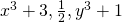Home » Uncategorized » Algebraic expression

# Algebraic expression

A combination of constant and variables connected by some or all at the the four fundamental operations, additions, substraction, multiplication & division is called an algabric expression
e.g- 3x + zy

Terms:- the different part of an algebraic expression separated by sign+ or – are called the terms of an expression.
e.g. 3x+2y (term – 3x & 2y)

factor of terms :- we can factroized all terms all terms.-
e.g 3x + 2y
3x = 3×x
2y = 2×y

Types of algebaric expressions.

Monomial– which contains only one term is said a monimial.
Ginomial– which contains two terms e.g. 3x + 2y
Trinomials– which contains three terms – e.g- 3x +2y +z
Quadrinomials – which contains four terms e.g. 2x + 3y + z-6
Polynomials– which contains one or more terms.

Degree of polynomials The highest power of the variable in a polynomials is called its degree.Here the degree of the polynomial is 3

Linear polynomial– a polynomial of degree is called a linear polynomials. E.g.- x+3

Quadratic polynomials– a polynomials of degree 2 is called a quadratic polynomials.

E.g. (x+2)(x+3) = x2 + 5x + 6

Cubic polynomial– A polynomial of degree 3 is called cubic polynomials.
e.g. degree of the term- 3x =1
2xy = 1+1 = 2
3x2g = 2 = 1 = 3

Algebraic expressions contains one or more forms and each terms contain variable and numerical coefficient, we find the value of terms to put the value of variables.
Equation– a statement of equality which invader one or more variable is called an equation. Terms of left hand side is equal to right hand side e.g – 3x+5 = 8

Solution of an equation e.g. 3x+5=8

(1 )Trial & error method– put the value of variable x, so that L.H.S= R.H.S.
Put the value of x= 1,2,3 who satisfy by the equation
3(1)=5 =8 the value of x= 1 is satisfied equation

(2) (A) if same number or terms is added, substract multiply or divide to both side of equation , the equation remain same (elemination method)
3x+5=8
3x=3(deduct 5 in both side)
X=1 (divid from 3 in both side)
(B) change in the side of required terms.
3x+5= 8
3x= 8-5
3x=3
x = 3/3 = 1

Managed by MochaHost - Fast & Easy WordPress Hosting